# Machine Learning 2 D 5362 Lecture 2 Concept

• Slides: 31Machine Learning 2 D 5362 Lecture 2: Concept Learning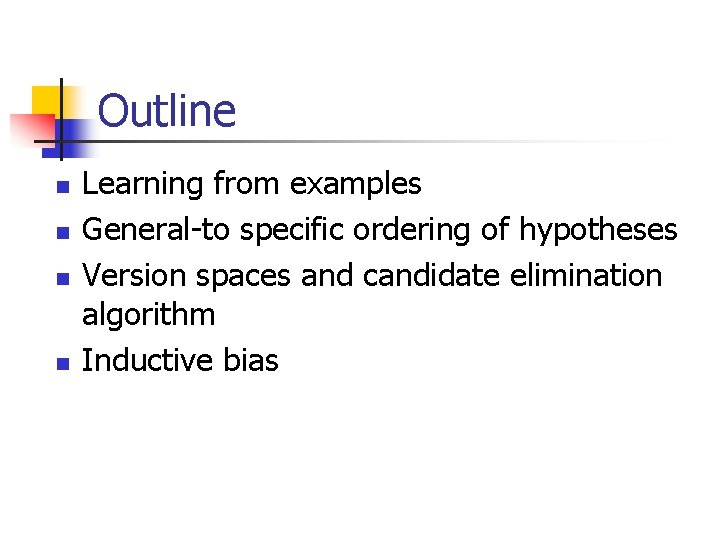Outline n n Learning from examples General-to specific ordering of hypotheses Version spaces and candidate elimination algorithm Inductive bias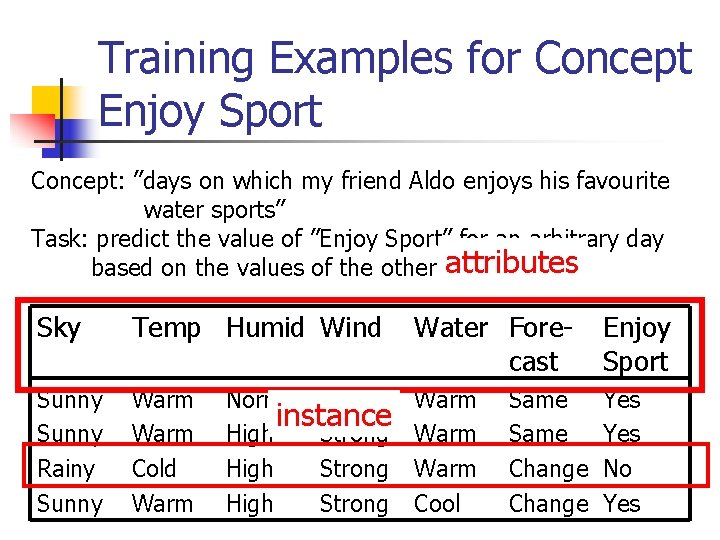Training Examples for Concept Enjoy Sport Concept: ”days on which my friend Aldo enjoys his favourite water sports” Task: predict the value of ”Enjoy Sport” for an arbitrary day attributes based on the values of the other attributes Sky Temp Humid Wind Water Forecast Enjoy Sport Sunny Rainy Sunny Warm Cold Warm Cool Yes Normal Strong instance High Strong Same ChangeRepresenting Hypothesis n n Hypothesis h is a conjunction of constraints on attributes Each constraint can be: n n n A specific value : e. g. Water=Warm A don’t care value : e. g. Water=? No value allowed (null hypothesis): e. g. Water=Ø Example: hypothesis h Sky Temp Humid Wind Water Forecast < Sunny ? ? Strong ? Same > n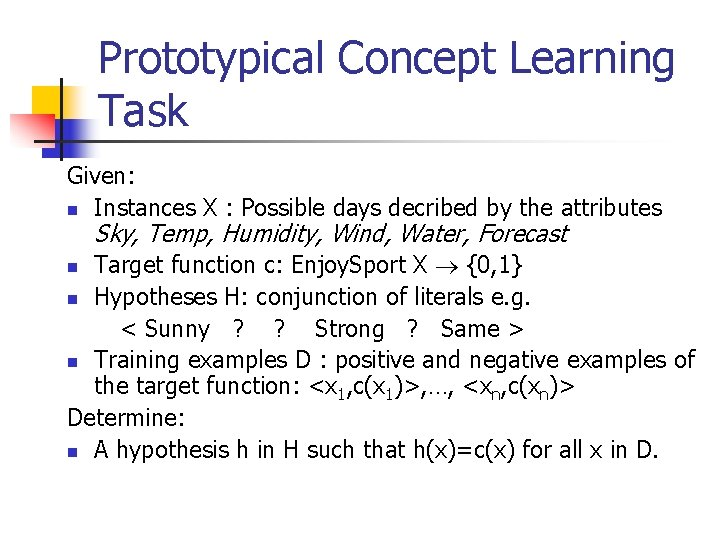Prototypical Concept Learning Task Given: n Instances X : Possible days decribed by the attributes Sky, Temp, Humidity, Wind, Water, Forecast Target function c: Enjoy. Sport X {0, 1} n Hypotheses H: conjunction of literals e. g. < Sunny ? ? Strong ? Same > n Training examples D : positive and negative examples of the target function: <x 1, c(x 1)>, …, <xn, c(xn)> Determine: n A hypothesis h in H such that h(x)=c(x) for all x in D. nInductive Learning Hypothesis n Any hypothesis found to approximate the target function well over the training examples, will also approximate the target function well over the unobserved examples.Number of Instances, Concepts, Hypotheses Sky: Sunny, Cloudy, Rainy n Air. Temp: Warm, Cold n Humidity: Normal, High n Wind: Strong, Weak n Water: Warm, Cold n Forecast: Same, Change #distinct instances : 3*2*2*2 = 96 #distinct concepts : 296 #syntactically distinct hypotheses : 5*4*4*4=5120 #semantically distinct hypotheses : 1+4*3*3*3=973 n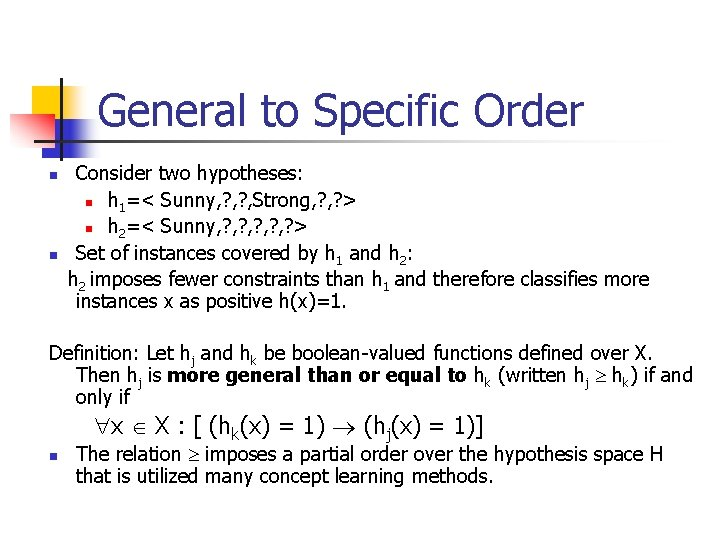General to Specific Order n n Consider two hypotheses: n h 1=< Sunny, ? , Strong, ? > n h 2=< Sunny, ? , ? , ? > Set of instances covered by h 1 and h 2: h 2 imposes fewer constraints than h 1 and therefore classifies more instances x as positive h(x)=1. Definition: Let hj and hk be boolean-valued functions defined over X. Then hj is more general than or equal to hk (written hj hk) if and only if x X : [ (hk(x) = 1) (hj(x) = 1)] n The relation imposes a partial order over the hypothesis space H that is utilized many concept learning methods.Instance, Hypotheses and ”more general” Instances Hypotheses specific x 1 h 3 x 2 h 2 h 1 h 2 h 3 h 1 h 2 general x 1=< Sunny, Warm, High, Strong, Cool, Same> h 1=< Sunny, ? , Strong, ? > x 2=< Sunny, Warm, High, Light, Warm, Same> h 2=< Sunny, ? , ? , ? > h 3=< Sunny, ? , ? , Cool, ? >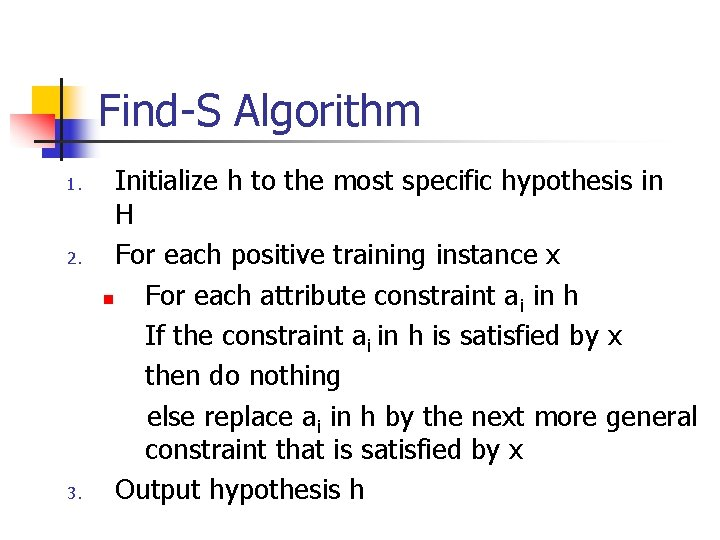Find-S Algorithm 1. 2. 3. Initialize h to the most specific hypothesis in H For each positive training instance x n For each attribute constraint ai in h If the constraint ai in h is satisfied by x then do nothing else replace ai in h by the next more general constraint that is satisfied by x Output hypothesis h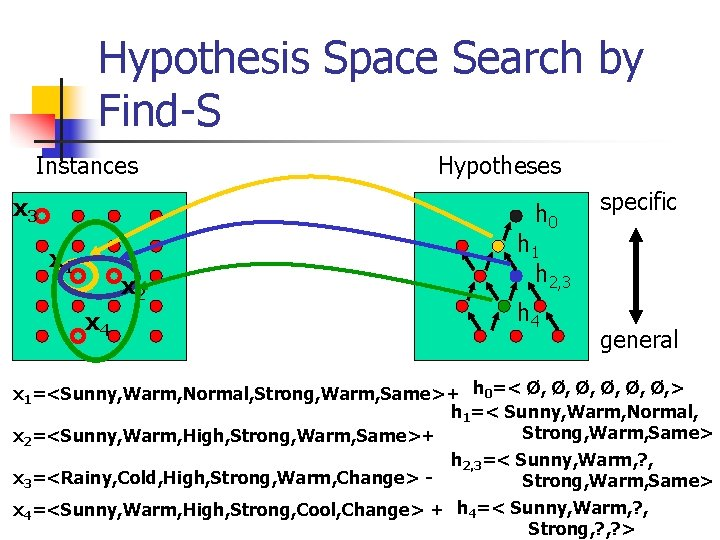Hypothesis Space Search by Find-S Instances x 3 Hypotheses h 0 x 1 x 2 x 4 specific h 1 h 2, 3 h 4 general x 1=<Sunny, Warm, Normal, Strong, Warm, Same>+ h 0=< Ø, Ø, Ø, > h 1=< Sunny, Warm, Normal, Strong, Warm, Same> x 2=<Sunny, Warm, High, Strong, Warm, Same>+ h 2, 3=< Sunny, Warm, ? , x 3=<Rainy, Cold, High, Strong, Warm, Change> Strong, Warm, Same> x 4=<Sunny, Warm, High, Strong, Cool, Change> + h 4=< Sunny, Warm, ? , Strong, ? >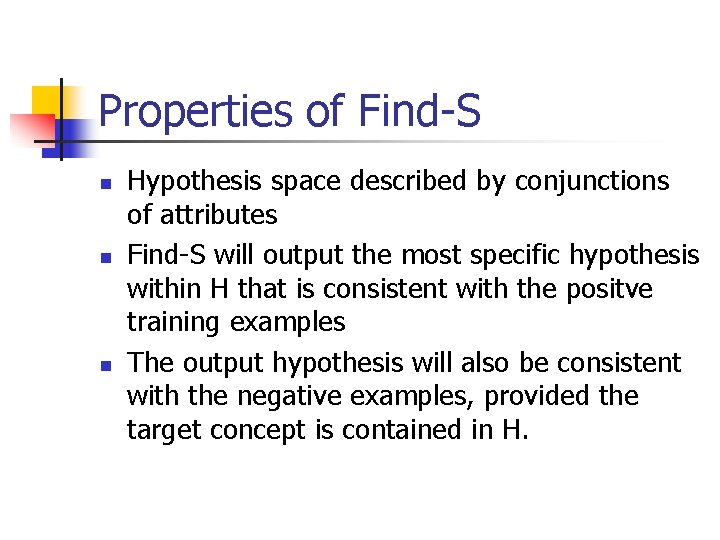Properties of Find-S n n n Hypothesis space described by conjunctions of attributes Find-S will output the most specific hypothesis within H that is consistent with the positve training examples The output hypothesis will also be consistent with the negative examples, provided the target concept is contained in H.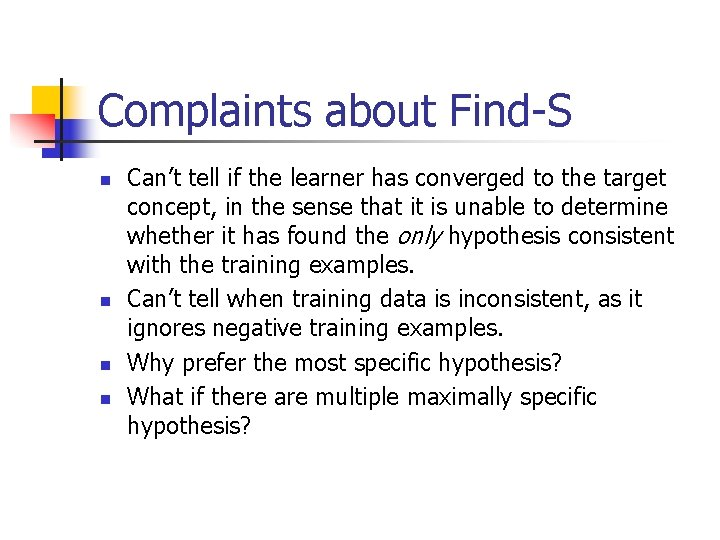Complaints about Find-S n n Can’t tell if the learner has converged to the target concept, in the sense that it is unable to determine whether it has found the only hypothesis consistent with the training examples. Can’t tell when training data is inconsistent, as it ignores negative training examples. Why prefer the most specific hypothesis? What if there are multiple maximally specific hypothesis?Version Spaces A hypothesis h is consistent with a set of training examples D of target concept if and only if h(x)=c(x) for each training example <x, c(x)> in D. Consistent(h, D) : = <x, c(x)> D h(x)=c(x) n The version space, VSH, D , with respect to hypothesis space H, and training set D, is the subset of hypotheses from H consistent with all training examples: VSH, D = {h H | Consistent(h, D) } n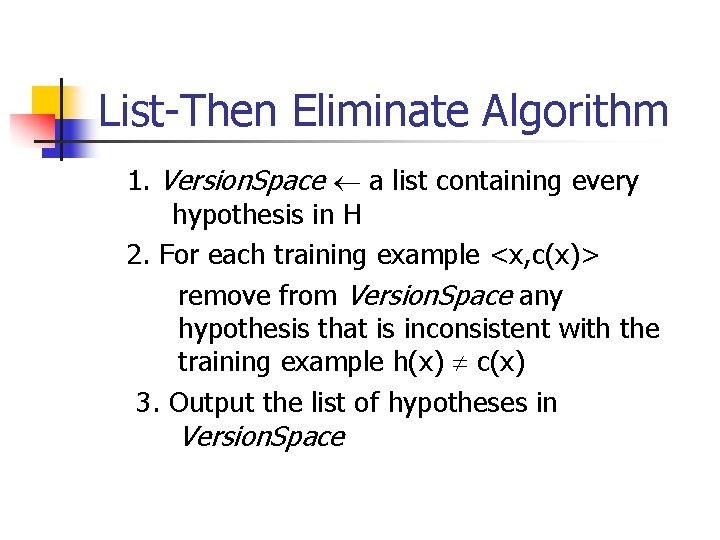List-Then Eliminate Algorithm 1. Version. Space a list containing every hypothesis in H 2. For each training example <x, c(x)> remove from Version. Space any hypothesis that is inconsistent with the training example h(x) c(x) 3. Output the list of hypotheses in Version. Space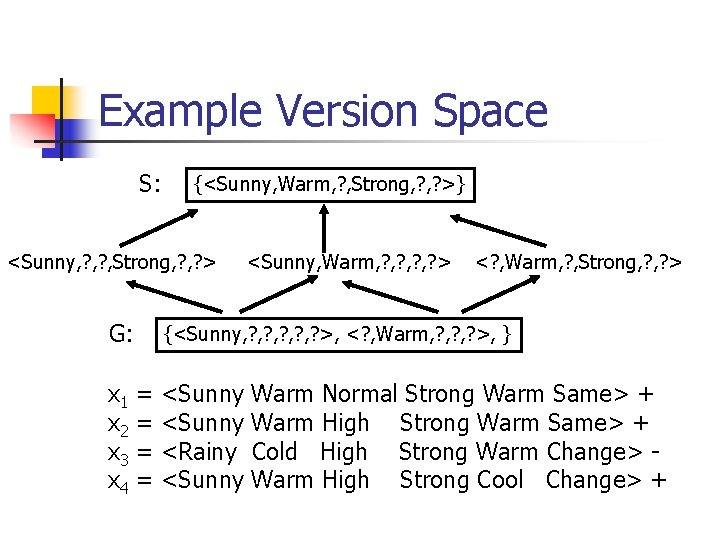Example Version Space S: {<Sunny, Warm, ? , Strong, ? >} <Sunny, ? , Strong, ? > G: x 1 x 2 x 3 x 4 <Sunny, Warm, ? , ? > <? , Warm, ? , Strong, ? > {<Sunny, ? , ? , ? >, <? , Warm, ? , ? >, } = = <Sunny <Rainy <Sunny Warm Normal Strong Warm Same> + Warm High Strong Warm Same> + Cold High Strong Warm Change> Warm High Strong Cool Change> +Representing Version Spaces n n n The general boundary, G, of version space VSH, D is the set of maximally general members. The specific boundary, S, of version space VSH, D is the set of maximally specific members. Every member of the version space lies between these boundaries VSH, D = {h H| ( s S) ( g G) (g h s) where x y means x is more general or equal than y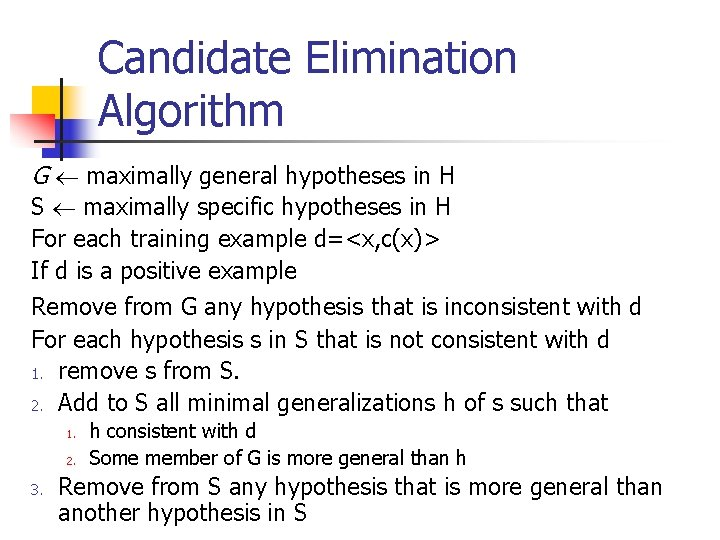Candidate Elimination Algorithm G maximally general hypotheses in H S maximally specific hypotheses in H For each training example d=<x, c(x)> If d is a positive example Remove from G any hypothesis that is inconsistent with d For each hypothesis s in S that is not consistent with d 1. remove s from S. 2. Add to S all minimal generalizations h of s such that 1. 2. 3. h consistent with d Some member of G is more general than h Remove from S any hypothesis that is more general than another hypothesis in S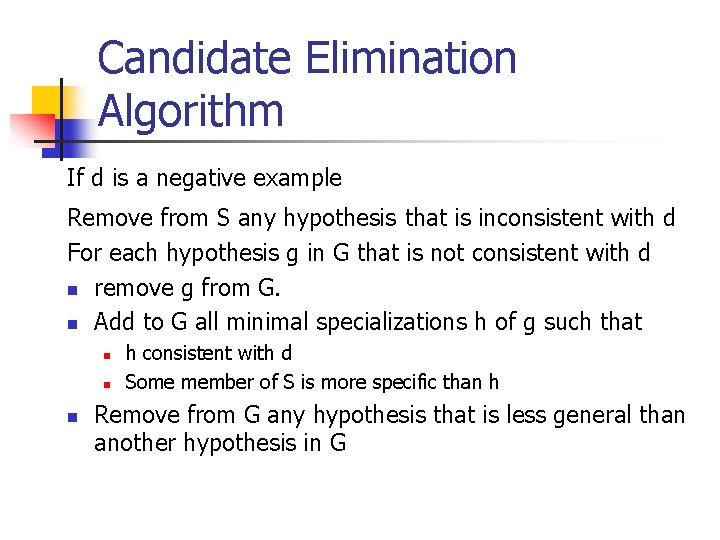Candidate Elimination Algorithm If d is a negative example Remove from S any hypothesis that is inconsistent with d For each hypothesis g in G that is not consistent with d n remove g from G. n Add to G all minimal specializations h of g such that n n n h consistent with d Some member of S is more specific than h Remove from G any hypothesis that is less general than another hypothesis in GExample Candidate Elimination S: {< , , , >} G: {<? , ? , ? , ? >} x 1 = <Sunny Warm Normal Strong Warm Same> + S: {< G: Sunny Warm Normal Strong Warm Same >} {<? , ? , ? , ? >} x 2 = <Sunny Warm High Strong Warm Same> + S: G: {< Sunny Warm ? Strong Warm Same >} {<? , ? , ? , ? >}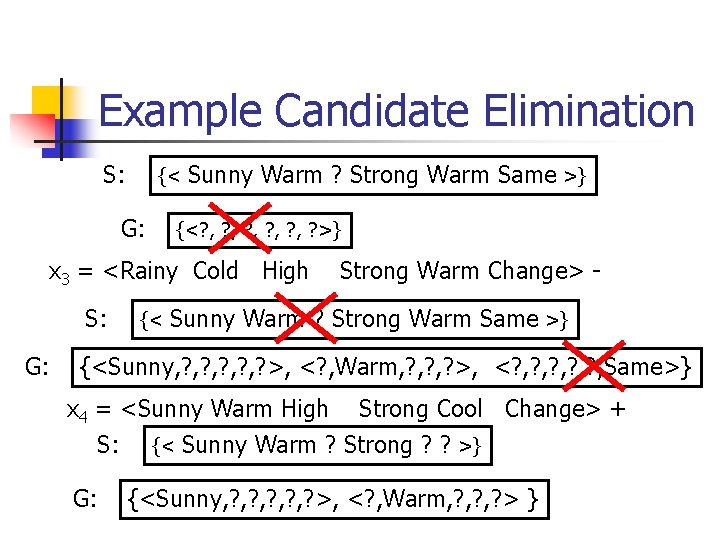Example Candidate Elimination S: {< G: Sunny Warm ? Strong Warm Same >} {<? , ? , ? , ? >} x 3 = <Rainy Cold High S: G: {< Strong Warm Change> - Sunny Warm ? Strong Warm Same >} {<Sunny, ? , ? , ? >, <? , Warm, ? , ? >, <? , ? , ? , Same>} x 4 = <Sunny Warm High Strong Cool Change> + S: {< Sunny Warm ? Strong ? ? >} G: {<Sunny, ? , ? , ? >, <? , Warm, ? , ? > }Example Candidate Elimination • Instance space: integer points in the x, y plane • hypothesis space : rectangles, that means hypotheses are of the form a x b , c y d. Homework: Exercise 2. 4Classification of New Data S: {<Sunny, Warm, ? , Strong, ? >} <Sunny, ? , Strong, ? > G: x 5 x 6 x 7 x 8 <Sunny, Warm, ? , ? > <? , Warm, ? , Strong, ? > {<Sunny, ? , ? , ? >, <? , Warm, ? , ? >, } = = <Sunny <Rainy <Sunny Warm Normal Strong Cool Change> + 6/0 Cold Normal Light Warm Same> - 0/6 Warm Normal Light Warm Same> ? 3/3 Cold Normal Strong Warm Same> ? 2/4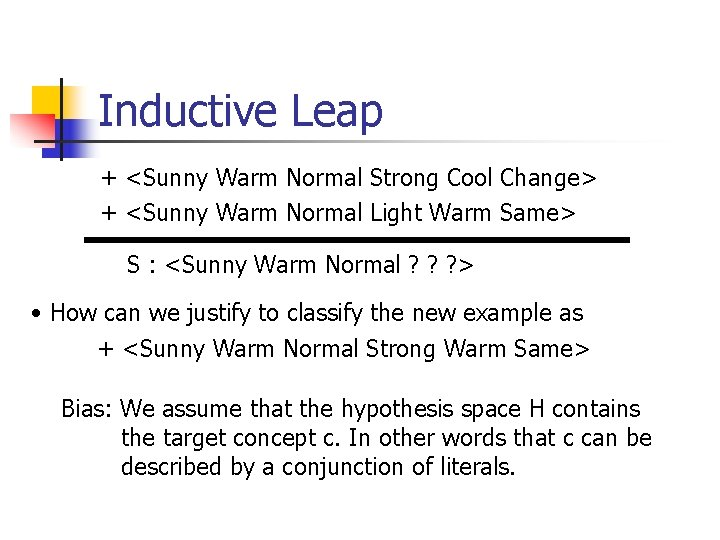Inductive Leap + <Sunny Warm Normal Strong Cool Change> + <Sunny Warm Normal Light Warm Same> S : <Sunny Warm Normal ? ? ? > • How can we justify to classify the new example as + <Sunny Warm Normal Strong Warm Same> Bias: We assume that the hypothesis space H contains the target concept c. In other words that c can be described by a conjunction of literals.Biased Hypothesis Space n Our hypothesis space is unable to represent a simple disjunctive target concept : (Sky=Sunny) v (Sky=Cloudy) x 1 = <Sunny Warm Normal Strong Cool Change> + x 2 = <Cloudy Warm Normal Strong Cool Change> + S : { <? , Warm, Normal, Strong, Cool, Change> } x 3 = <Rainy Warm Normal Light Warm Same> S : {}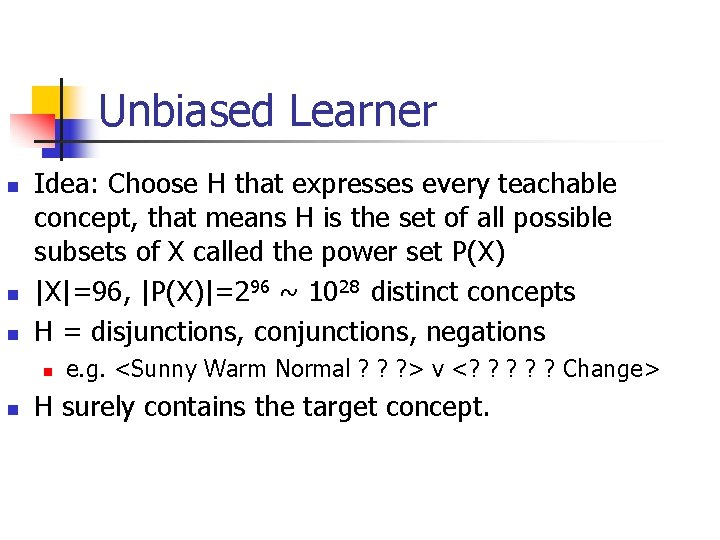Unbiased Learner n n n Idea: Choose H that expresses every teachable concept, that means H is the set of all possible subsets of X called the power set P(X) |X|=96, |P(X)|=296 ~ 1028 distinct concepts H = disjunctions, conjunctions, negations n n e. g. <Sunny Warm Normal ? ? ? > v <? ? ? Change> H surely contains the target concept.Unbiased Learner What are S and G in this case? Assume positive examples (x 1, x 2, x 3) and negative examples (x 4, x 5) S : { (x 1 v x 2 v x 3) } G : { (x 4 v x 5) } The only examples that are classified are the training examples themselves. In other words in order to learn the target concept one would have to present every single instance in X as a training example. Each unobserved instance will be classified positive by precisely half the hypothesis in VS and negative by the other half.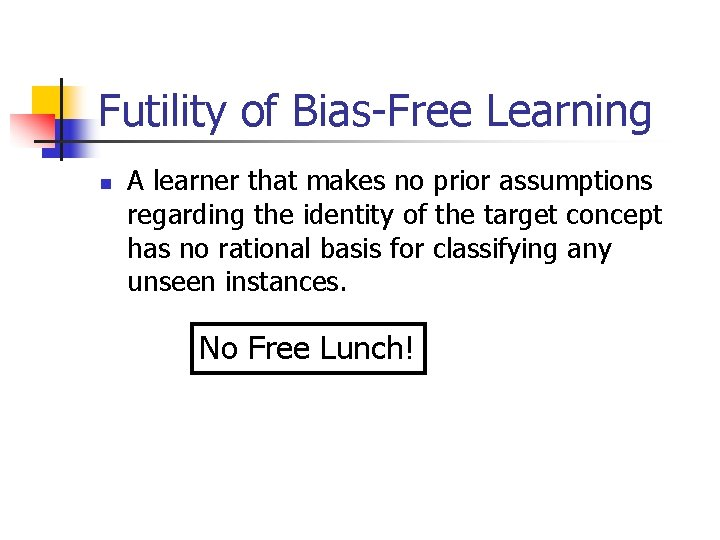Futility of Bias-Free Learning n A learner that makes no prior assumptions regarding the identity of the target concept has no rational basis for classifying any unseen instances. No Free Lunch!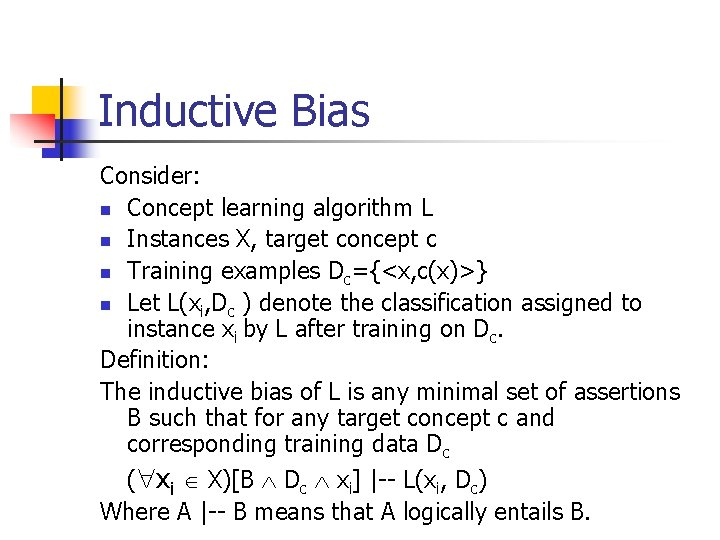Inductive Bias Consider: n Concept learning algorithm L n Instances X, target concept c n Training examples Dc={<x, c(x)>} n Let L(xi, Dc ) denote the classification assigned to instance xi by L after training on Dc. Definition: The inductive bias of L is any minimal set of assertions B such that for any target concept c and corresponding training data Dc ( xi X)[B Dc xi] |-- L(xi, Dc) Where A |-- B means that A logically entails B.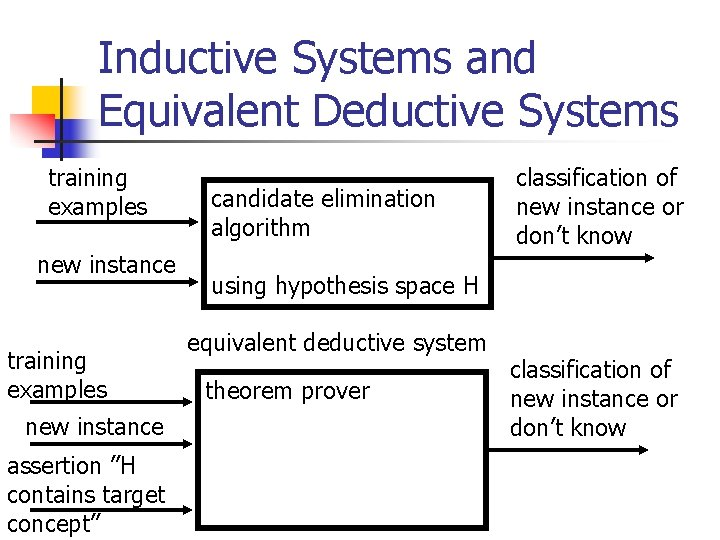Inductive Systems and Equivalent Deductive Systems training examples new instance assertion ”H contains target concept” candidate elimination algorithm classification of new instance or don’t know using hypothesis space H equivalent deductive system theorem prover classification of new instance or don’t know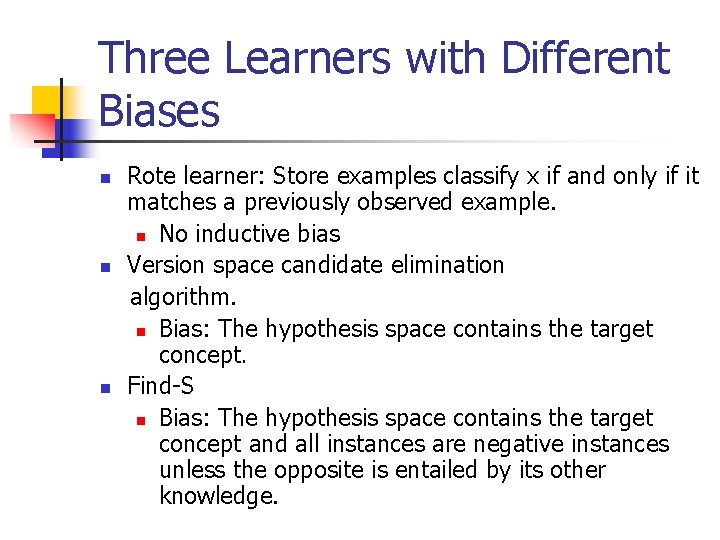Three Learners with Different Biases n n n Rote learner: Store examples classify x if and only if it matches a previously observed example. n No inductive bias Version space candidate elimination algorithm. n Bias: The hypothesis space contains the target concept. Find-S n Bias: The hypothesis space contains the target concept and all instances are negative instances unless the opposite is entailed by its other knowledge.